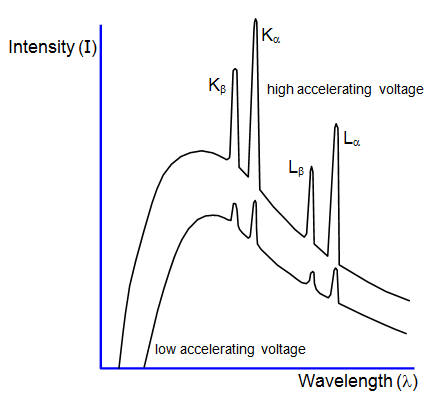# X-ray spectraUsing X-ray diffraction the spectrum produced by an X-ray tube can be investigated. Such a spectrum is shown in the diagram, in which the two curves represent two different accelerating voltages.

The X-ray spectrum can be considered in two parts:
(a) a continuous background, and (b) a series of sharp peaks.

The background is due to radiation emitted as the electrons are slowed down by electromagnetic attraction of the nuclei of the material. The minimum wavelength and therefore the maximum energy and frequency of an X-ray in this spectrum is produced when an electron is stopped by just one nucleus. The rest of the curve is produced by electrons losing only part of their energy during collisions with many nuclei. The minimum wavelength λm is given by the equation:

Minimum X ray wavelength (λm) = hc/eV

where V is the accelerating voltage and c the velocity of light: the smaller the value of V, the greater the minimum wavelength will be and the smaller the maximum frequency.

The peaks on the spectrum are characteristic of the particular target material. Electrons from the inner shells are removed completely by the bombarding electrons. An electron from an outer energy level falls back to fill the vacancy emitting a photon of a definite wavelength, thus giving a sharp peak on the spectrum.

A VERSION IN WORD IS AVAILABLE ON THE SCHOOLPHYSICS USB

© Keith Gibbs 2020